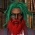Display replies flat, with oldest first Display replies flat, with newest first Display replies in threaded form Display replies in nested formМиникурс лаб Чебышева: Enzo Orsingher, "Fractional calculus and applications to stochastic processes"by Egor Pifagorov - Monday, 12 March 2012, 08:34 PM Fractional calculus and applications to stochastic processes PROF. ENZO ORSINGHER 24.03, 26.03, 28.03, 31.03, 02.04, 04.04 17-00, 14-ая линия, ауд. 14 (в первый раз!) PROGRAM OF THE MINICOURSE 1. Introduction to fractional calculus The Riemann-Liouville integral. The Riemann-Liouville derivative. Relationship between fractional derivative and fractional integral and between fractional integral and fractional derivative. The Marchaud derivative. Laplace transform of fractional derivative. Dzhrbashyan-Caputo derivative. Laplace transform of the Dzhrbashyan-Caputo derivative. 2. Fractional diffusion equation Mittag-Leffler function. Wright functions. Solution of the fractional di usion equation. 3. Riesz calculus The Riesz integral. The Riesz derivative. Space-fractional equation and stable processes. 4. Fractional Poisson process Construction and distribution. Time-fractional Poisson process as a renewal process. 5. Fractional pure birth process: non-linear and linear case 6. Fractional telegraph equation: solution and discussion Some time will be devoted at the beginnig to the most important results on Gamma function.